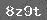# 小学奥数知识点趣味学习 —— 相遇问题

相遇问题

两个运动物体作相向运动或在环形跑道上作背向运动，随着时间的发展，必然面对面地相遇，这类问题叫做相遇问题。它的特点是两个运动物体共同走完整个路程。相遇问题根据数量关系可分成三种类型：求路程，求相遇时间，求速度。

它们的基本关系式如下：

总路程=$甲速+乙速$×相遇时间

相遇时间=总路程÷$甲速+乙速$

另一个速度=甲乙速度和-已知的一个速度

1

.求路程

$1$求两地间的距离

例1两辆汽车同时从甲、乙两地相对开出，一辆汽车每小时行56千米，另一辆汽车每小时行63千米，经过4小时后相遇。甲乙两地相距多少千米?$适于五年级程度$

解：两辆汽车从同时相对开出到相遇各行4小时。一辆汽车的速度乘以它行驶的时间，就是它行驶的路程;另一辆汽车的速度乘以它行驶的时间，就是这辆汽车行驶的路程。两车行驶路程之和，就是两地距离。

56×4=224$千米$

63×4=252$千米$

224+252=476$千米$

综合算式：

56×4+63×4

=224+252

=476$千米$

答略。

例2两列火车同时从相距480千米的两个城市出发，相向而行，甲车每小时行驶40千米，乙车每小时行驶42千米。5小时后，两列火车相距多少千米?$适于五年级程度$

解：此题的答案不能直接求出，先求出两车5小时共行多远后，从两地的距离480千米中，减去两车5小时共行的路程，所得就是两车的距离。

480-$40+42$×5

=480-82×5

=480-410

=70$千米$

答：5小时后两列火车相距70千米。

例3两列火车从甲、乙两地同时出发对面开来，第一列火车每小时行驶60千米，第二列火车每小时行驶55千米。两车相遇时，第一列火车比第二列火车多行了20千米。求甲、乙两地间的距离。$适于五年级程度$

解：两车相遇时，两车的路程差是20千米。出现路程差的原因是两车行驶的速度不同，第一列火车每小时比第二列火车多行$60-55$千米。由此可求出两车相遇的时间，进而求出甲、乙两地间的距离。

$60+55$×[20÷$60-55$]

=115×[20÷5]

=460$千米$

答略。

2

.求相遇时间

例1两个城市之间的路程是500千米，一列客车和一列货车同时从两个城市相对开出，客车的平均速度是每小时55千米，货车的平均速度是每小时45千米。两车开了几小时以后相遇?$适于五年级程度$

解：已知两个城市之间的路程是500千米，又知客车和货车的速度，可求出两车的速度之和。用两城之间的路程除以两车的速度之和可以求出两车相遇的时间。

500÷$55+45$

=500÷100

=5$小时$

答略。

例2在一次战役中，敌我双方原来相距62.75千米。据侦察员报告，敌人已向我处前进了11千米。我军随即出发迎击，每小时前进6.5千米，敌人每小时前进5千米。我军出发几小时后与敌人相遇?$适于五年级程度$

解：此题已给出总距离是62.75千米，由“敌人已向我处前进了11千米”可知实际的总距离减少到$62.75-11$千米。

$62.75-11$÷$6.5+5$

=51.75÷11.5

=4.5$小时$

答：我军出发4.5小时后与敌人相遇。

例3甲、乙两地相距200千米，一列货车由甲地开往乙地要行驶5小时;一列客车由乙地开往甲地需要行驶4小时。如果两列火车同时从两地相对开出，经过几小时可以相遇?$得数保留一位小数$$适于五年级程度$

解：此题用与平常说法不同的方式给出了两车的速度。先分别求出速度再求和，根据“时间=路程÷速度”的关系，即可求出相遇时间。

200÷$200÷5+200÷4$

=200÷$40+50$

=200÷90

≈2.2$小时$

答：两车大约经过2.2小时相遇。

例4在复线铁路上，快车和慢车分别从两个车站开出，相向而行。快车车身长是180米，速度为每秒钟9米;慢车车身长210米，车速为每秒钟6米。从两车头相遇到两车的尾部离开，需要几秒钟?$适于五年级程度$

解：因为是以两车离开为准计算时间，所以两车经过的路程是两个车身的总长。总长除以两车的速度和，就得到两车从相遇到车尾离开所需要的时间。

$180+210$÷$9+6$

=390÷15

=26$秒$

答略。

3

求速度

例1甲、乙两个车站相距550千米，两列火车同时由两站相向开出，5小时相遇。快车每小时行60千米。慢车每小时行多少千米?$适于五年级程度$

解：先求出速度和，再从速度和中减去快车的速度，便得出慢车每小时行：

550÷5-60

=110-60

=50$千米$

答略。

例2A、B两个城市相距380千米。客车和货车从两个城市同时相对开出，经过4小时相遇。货车比客车每小时快5千米。这两列车每小时各行多少千米?$适于五年级程度$

解：客车每小时行：

$380÷4-5$÷2

=$95-5$÷2

=45$千米$

货车每小时行：

45+5=50$千米$

答略。

例3甲、乙两个城市相距980千米，两列火车由两城市同时相对开出，经过10小时相遇。快车每小时行50千米，比慢车每小时多行多少千米?$适于五年级程度$

解：两城市的距离除以两车相遇的时间，得到两车的速度和。从两车的速度和中减去快车的速度，得到慢车的速度。再用快车速度减去慢车的速度，即得到题中所求。

50-$980÷10-50$

=50-$98-50$

=50-48

=2$千米$

答略。

### 相关推荐

 留言与评论（共有 0 条评论）

 验证码：